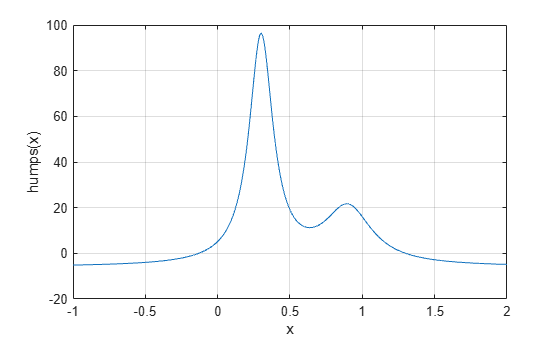# Roots of Scalar Functions

### Solving a Nonlinear Equation in One Variable

The `fzero` function attempts to find a root of one equation with one variable. You can call this function with either a one-element starting point or a two-element vector that designates a starting interval. If you give `fzero` a starting point `x0`, `fzero` first searches for an interval around this point where the function changes sign. If the interval is found, `fzero` returns a value near where the function changes sign. If no such interval is found, `fzero` returns `NaN`. Alternatively, if you know two points where the function value differs in sign, you can specify this starting interval using a two-element vector; `fzero` is guaranteed to narrow down the interval and return a value near a sign change.

The following sections contain two examples that illustrate how to find a zero of a function using a starting interval and a starting point. The examples use the function `humps.m`, which is provided with MATLAB®. The following figure shows the graph of `humps`.

```x = -1:.01:2; y = humps(x); plot(x,y) xlabel('x'); ylabel('humps(x)') grid on```### Setting Options For `fzero`

You can control several aspects of the `fzero` function by setting options. You set options using `optimset`. Options include:

### Using a Starting Interval

The graph of `humps` indicates that the function is negative at `x = -1` and positive at `x = 1`. You can confirm this by calculating `humps` at these two points.

`humps(1)`
```ans = 16 ```
`humps(-1)`
```ans = -5.1378 ```

Consequently, you can use `[-1 1]` as a starting interval for `fzero`.

The iterative algorithm for `fzero` finds smaller and smaller subintervals of `[-1 1]`. For each subinterval, the sign of `humps` differs at the two endpoints. As the endpoints of the subintervals get closer and closer, they converge to zero for `humps`.

To show the progress of `fzero` at each iteration, set the `Display` option to `iter` using the `optimset` function.

`options = optimset('Display','iter');`

Then call `fzero` as follows:

`a = fzero(@humps,[-1 1],options)`
``` Func-count x f(x) Procedure 2 -1 -5.13779 initial 3 -0.513876 -4.02235 interpolation 4 -0.513876 -4.02235 bisection 5 -0.473635 -3.83767 interpolation 6 -0.115287 0.414441 bisection 7 -0.115287 0.414441 interpolation 8 -0.132562 -0.0226907 interpolation 9 -0.131666 -0.0011492 interpolation 10 -0.131618 1.88371e-07 interpolation 11 -0.131618 -2.7935e-11 interpolation 12 -0.131618 8.88178e-16 interpolation 13 -0.131618 8.88178e-16 interpolation Zero found in the interval [-1, 1] ```
```a = -0.1316 ```

Each value `x` represents the best endpoint so far. The `Procedure` column tells you whether each step of the algorithm uses bisection or interpolation.

You can verify that the function value at `a` is close to zero by entering

`humps(a)`
```ans = 8.8818e-16 ```

### Using a Starting Point

Suppose you do not know two points at which the function values of `humps` differ in sign. In that case, you can choose a scalar `x0` as the starting point for `fzero`. `fzero` first searches for an interval around this point on which the function changes sign. If `fzero` finds such an interval, it proceeds with the algorithm described in the previous section. If no such interval is found, `fzero` returns `NaN`.

For example, set the starting point to `-0.2`, the `Display` option to `Iter`, and call `fzero`:

```options = optimset('Display','iter'); a = fzero(@humps,-0.2,options)```
``` Search for an interval around -0.2 containing a sign change: Func-count a f(a) b f(b) Procedure 1 -0.2 -1.35385 -0.2 -1.35385 initial interval 3 -0.194343 -1.26077 -0.205657 -1.44411 search 5 -0.192 -1.22137 -0.208 -1.4807 search 7 -0.188686 -1.16477 -0.211314 -1.53167 search 9 -0.184 -1.08293 -0.216 -1.60224 search 11 -0.177373 -0.963455 -0.222627 -1.69911 search 13 -0.168 -0.786636 -0.232 -1.83055 search 15 -0.154745 -0.51962 -0.245255 -2.00602 search 17 -0.136 -0.104165 -0.264 -2.23521 search 18 -0.10949 0.572246 -0.264 -2.23521 search Search for a zero in the interval [-0.10949, -0.264]: Func-count x f(x) Procedure 18 -0.10949 0.572246 initial 19 -0.140984 -0.219277 interpolation 20 -0.132259 -0.0154224 interpolation 21 -0.131617 3.40729e-05 interpolation 22 -0.131618 -6.79505e-08 interpolation 23 -0.131618 -2.98428e-13 interpolation 24 -0.131618 8.88178e-16 interpolation 25 -0.131618 8.88178e-16 interpolation Zero found in the interval [-0.10949, -0.264] ```
```a = -0.1316 ```

The endpoints of the current subinterval at each iteration are listed under the headings `a` and `b`, while the corresponding values of `humps` at the endpoints are listed under `f(a)` and `f(b)`, respectively.

Note: The endpoints `a` and `b` are not listed in any specific order: `a` can be greater than `b` or less than `b`.

For the first nine steps, the sign of `humps` is negative at both endpoints of the current subinterval, which is shown in the output. At the tenth step, the sign of `humps` is positive at `a`, `-0.10949`, but negative at `b`, `-0.264`. From this point on, the algorithm continues to narrow down the interval `[-0.10949 -0.264]`, as described in the previous section, until it reaches the value `-0.1316`.